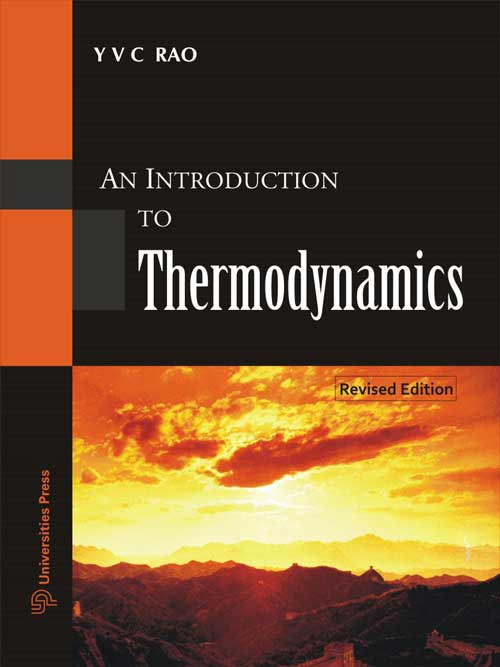## Introduction to Thermodynamics, An

#### Price: 965.00

This book provides a precise and logical presentation of the concepts and principles of thermodynamics from the macroscopic (classical) point of view. In addition to the nice presentation of theory, each chapter of the book has many solved examples which will help the students in better understanding the basic concepts. Further, several problems are given at the end of each chapter, with answers at the end of the book, for the students to solve by applying thermodynamic principles.

Preface
Nomenclature
Introduction
Concepts and Definitions
Thermodynamics Properties of Fluids
Zeroth Law of Thermodynamics
First Law of Thermodynamics
First Law Analysis of Processes
Second Law of Thermodynamics
Thermodynamics
Power and Refrigeration Cycles
Gas-Vapor Mixtures and Psychrometry
Appendices